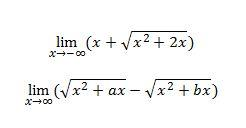# Limits

## Recommended Posts

I'm having problems with 2 limits:

I did the first, and I got undefined (to be precise, 2/(1 -1))

My TI-89, and the textbook, say that the result is -1.

On the second, I have no idea how to do it.

My TI-89, and the textbook, say that the result is a/2 - b/2 or 1/2(a-b).

Any thoughts? I would appreciate the processesEdited by josh24
##### Share on other sites

I don't know if this is a kosher way to do it, but the simplest way would be to add a constant to your square root to make it a perfect square. But then you have to prove adding the constant doesn't change the answer at the limit.

##### Share on other sites

I'm having problems with 2 limits:

I did the first, and I got undefined (to be precise, 2/(1 -1))

My TI-89, and the textbook, say that the result is -1.

On the second, I have no idea how to do it.

My TI-89, and the textbook, say that the result is a/2 - b/2 or 1/2(a-b).

Any thoughts? I would appreciate the processesThe first one obviously grows without bound as increases.

For the second one, rewrite it as something with an infinite limit times something with a zero limit then as a quotient of two expressions with zero limit and apply l'Hopital's rule. Burn your TI-89 and replace it with thought.

##### Share on other sites

The first one obviously grows without bound as increases.

I think you misread that. In the first limit, x is decreasing to negative infinity, not increasing to infinity. I'm not certain of how to solve it myself (I really need to brush up, apparently), but going through the first several negative integers it does seem to be going to -1 after being non-real on (-2,0).

##### Share on other sites

For the first answer could you not expand square root

ignoring coefficiants after first two terms

$\sqrt{(x^2+2x)} \approx {(x^2)}^{1/2} + \frac{x}{{(x^2)}^{1/2}} - \frac{x^2}{(x^2)^{3/2}} + \frac{x^3}{(x^2)^{5/2}} .....$

I have left them unsimplified to avoid losing the sign when squaring and then rooting. The terms of the xpansion after the second all tend to zero as x gets very large in magnitude (they have an increasing power of x in the denominator) . So

$x+ \sqrt{(x^2+2x)} \approx x + {(x^2)}^{1/2} + \frac{x}{{(x^2)}^{1/2}}$

If [imath] x [/imath] is negative then it is clear that the first term of the expansion ie [imath] {(x^2)}^{1/2} [/imath] will cancel the [imath] x [/imath] - which is why I didn't simplify [imath] {(x^2)}^{1/2} [/imath] to [imath] x [/imath]

and as the first term cancels with the x then all you are left with is the second term. When [imath] x [/imath] is negative it is clear that [imath]\frac{x}{{(x^2)}^{1/2}} [/imath] is equal to [imath] -1[/imath]

##### Share on other sites

I think you misread that. In the first limit, x is decreasing to negative infinity, not increasing to infinity. I'm not certain of how to solve it myself (I really need to brush up, apparently), but going through the first several negative integers it does seem to be going to -1 after being non-real on (-2,0).

You are right. I missed the minus sign.

You can do that one using the hint I gave for the second limit.

##### Share on other sites

• 4 weeks later...

I'm having problems with 2 limits:

I did the first, and I got undefined (to be precise, 2/(1 -1))

My TI-89, and the textbook, say that the result is -1.

On the second, I have no idea how to do it.

My TI-89, and the textbook, say that the result is a/2 - b/2 or 1/2(a-b).

Any thoughts? I would appreciate the processesObviously the -1 limit is of the :$lim_{n\to\infty}x-\sqrt{x^2+2x}$ ,which you will get,if you multiply by the : $\frac{x+\sqrt{x^2+2x}}{x+\sqrt{x^2+2x}}$ fraction,then divide numerator and denominator by x and then take the limit

For the other limit do exactly the same and the result will be :$\frac{a-b}{2}$

## Create an account

Register a new account